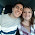## Saturday, February 26, 2011

### PHP Code Sample : Convert GPS / NMEA Lat Long to Decimal Degrees

This piece of code converts NMEA/GPS Lat -Long to Decimal Degrees. Code comments are self explanatory. The code is not graceful, but it works.
`function degree2decimal(\$deg_coord){//reference http://www.directionsmag.com/site/latlong-converter//GPS/NMEA fixes are in Degree Minutes.m format//for Google maps we need to convert them to decimal degrees//sample format of GPS  4533.35 is 45 degrees and 33.35 minutes//formula is as follows//Degrees=Degrees //.d = M.m/60//Decimal Degrees=Degrees+.d\$degree=(int)(\$deg_coord/100); //simple way\$minutes= \$deg_coord-(\$degree*100);\$dotdegree=\$minutes/60;\$decimal=\$degree+\$dotdegree;\$direction=substr(\$deg_coord,-1);//South latitudes and West longitudes need to return a negative resultif ((\$direction=="S") or (\$direction=="W"))        { \$decimal=\$decimal*(-1);}\$decimal=number_format(\$decimal,4,'.',''); //truncate decimal to 4 placesreturn \$decimal;}`

1.2.3.4.